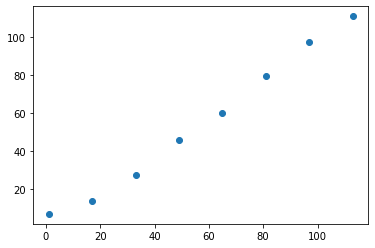# Ivory Documentation

Ivory is a lightweight framework for machine learning. It integrates model design, tracking, and hyperparmeter tuning. Ivory uses MLflow Tracking for tracking and Optuna for hyperparmeter tuning.

Using Ivory, you can tackle both tracking and tuning workflow at one place.

Another key feature of Ivory is its workflow design. You can write down all of your workflow such as model structure or tracking/tuning process in one YAML file. It allows us to understand the whole process at a glance.

Ivory is library-agnostic. You can use it with any machine learning library.

Get started using the Quickstart.

Or take a look at the code below.

``````import numpy as np

from ivory.callbacks.results import Results
from ivory.core.data import Data, Dataset, Datasets
from ivory.core.run import Run
from ivory.sklearn.estimator import Estimator
from ivory.sklearn.metrics import Metrics

data = Data()
data.index = np.arange(30)
data.input = np.arange(60).reshape(30, -1)
data.target = np.sum(data.input, axis=1)
data.fold = data.index % 4
datasets = Datasets(data, Dataset, fold=0)

estimator = Estimator(
model='sklearn.ensemble.RandomForestRegressor',
n_estimators=10,
max_depth=5,
)

run = Run(
name='first example',
datasets=datasets,
estimator=estimator,
results=Results(),
metrics=Metrics()
)
run.start()``````

 2020-06-20 15:23:32 (417ms) python3 (431ms)

``[first example] mse=14.7``
``````import matplotlib.pyplot as plt

plt.scatter(run.results.val.target, run.results.val.output)``````

 2020-06-20 15:23:33 (242ms) python3 (673ms)

``<matplotlib.collections.PathCollection at 0x140448ad408>``